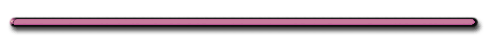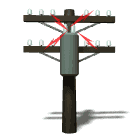Temperature and Current - MIT TV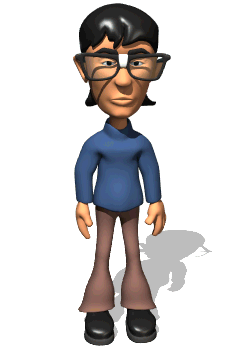"Why does the bulb get brighter when the rod is put in the liquid nitrogen?""Lowering the temperature of a wire  reduces resistance and increases current"

II. Circuit Concepts

Conservation of charge and energy for electric current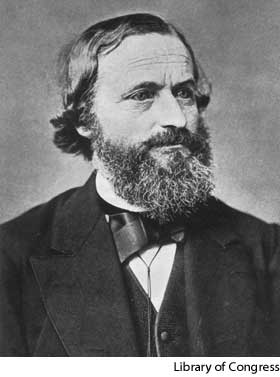Gustav Robert Kirchhoff
(March 12, 1824 – October 17, 1887) was a German physicist

This image is in the public domain because its copyright has expired.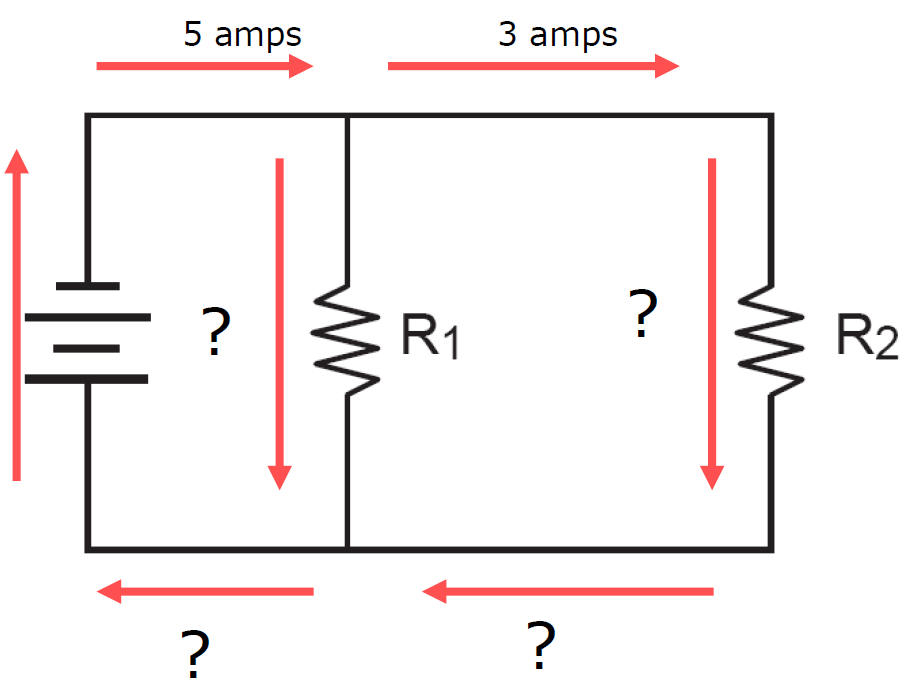1) Kirchhoff's 1st Law

 Total current arriving at a point in a circuit =   Total current leaving the  point. (Conservation of Charge)

“Amps In = Amps Out”Ex 1)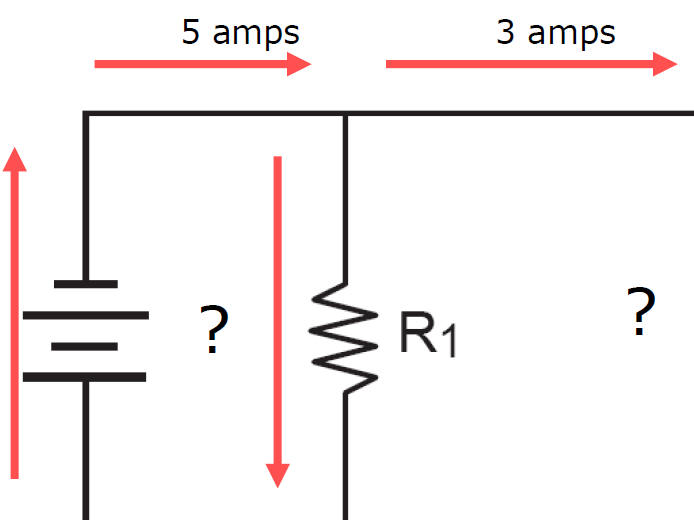Amps In = Amps Out

5 Amps = 3 Amps + x

X = 2 ampsRemember

“Amps In =
Amps Out”Ex 2) Current in bottom branch?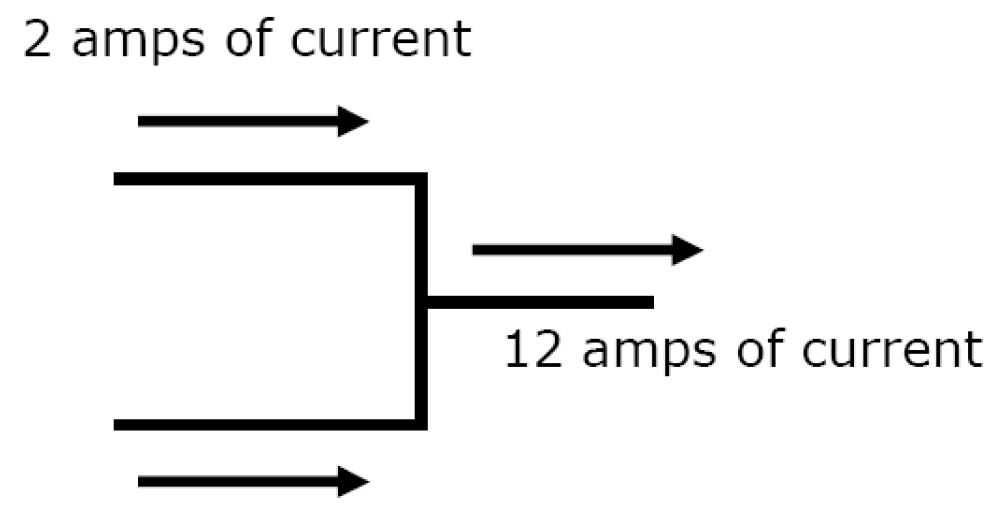Amps In = Amps Out

 2 Amps + X = 12 Amps

X = 10 ampsEx 3)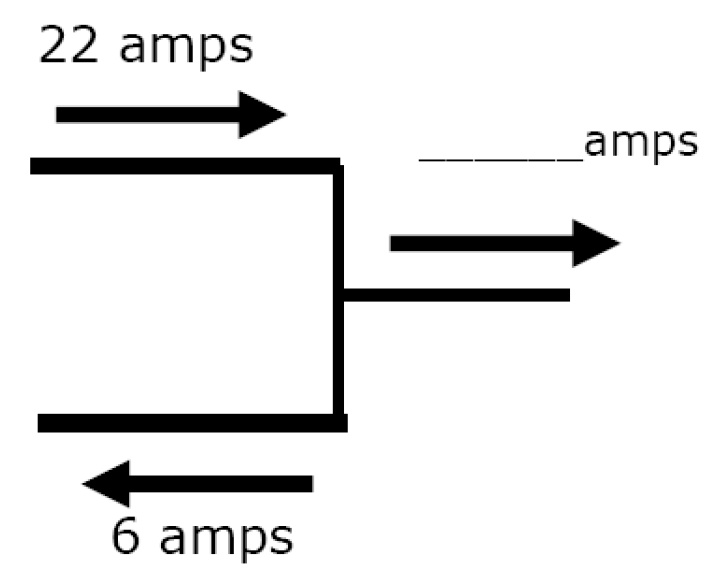Amps In = Amps Out

 22 Amps = x Amps + 6 Amps

X = 16 ampsEx 4)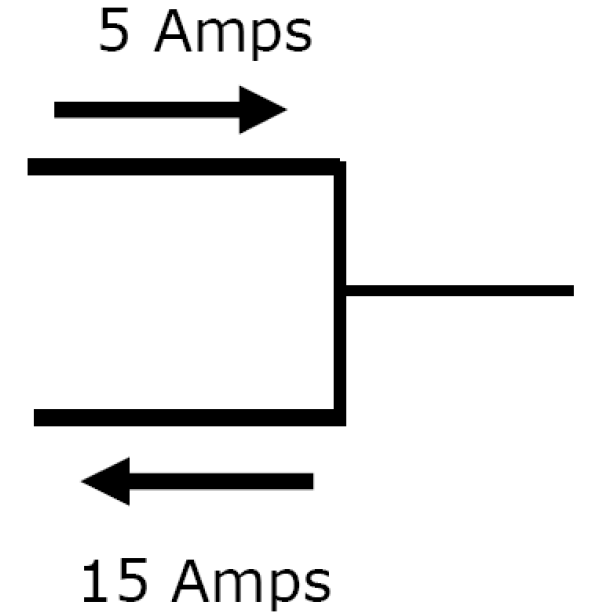Amps In = Amps Out

5 A + x = 15 A

x = 10 A INWhat's Wrong With This Picture?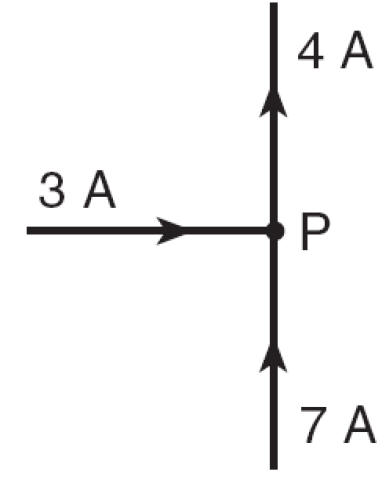Current In (10 A), does not equal current out (4 A)2) Kirchhoff's 2nd Law - the algebraic sum of all the voltage drops and applied voltages around a circuit = 0

(conservation of energy)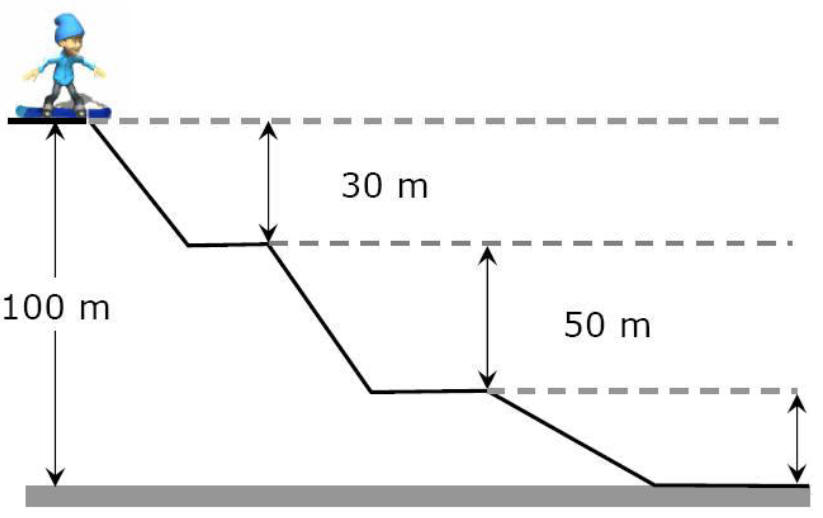Amount of PE gained when you go up a ski slope = energy released going down a ski slope

(KE, Work against friction and snow)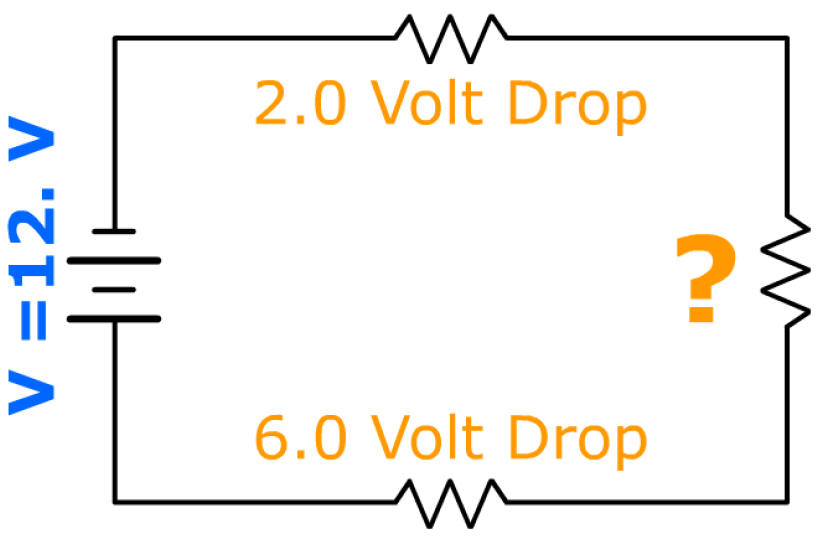Applied Voltage ( + )
Voltage Drop
( - )

0 =  12 V + -2 V + x + -6 V

0 = 12 V + -8 V + x

0 = 4 V + x

x = - 4 volts

 VT = V1  + V2 + ...